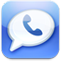﻿ The definition to Square Foot and Linear Foot
Top FAQ

### The definition to Square Foot and Linear Foot

2017-02-20Tags:Square, Linear,Foot,Posted By zhaozhao

The definition to Square Foot and Linear Foot

A square foot is a unit of measurement of area. A piece of material measuring 12 inches by 12 inches is one square foot. To compute the number of square feet in a piece of wire mesh you multiply its width in feet times its length in feet. For example, a piece of wire mesh 4 feet wide and 3 feet long would be 12 square feet.

Most wire mesh is priced by the square foot.

A linear foot is a unit of measurement of length. A piece of material measuring, for example, 3 feet wide by 15 feet long would be 15 linear feet. The same piece of material could be described as 45 square feet; 3 feet wide X 15 linear feet which equals 45 square feet. Note: sometimes the term "running foot is used to mean linear foot".Tel